Robotics 1
Camera Coordinates
Quiz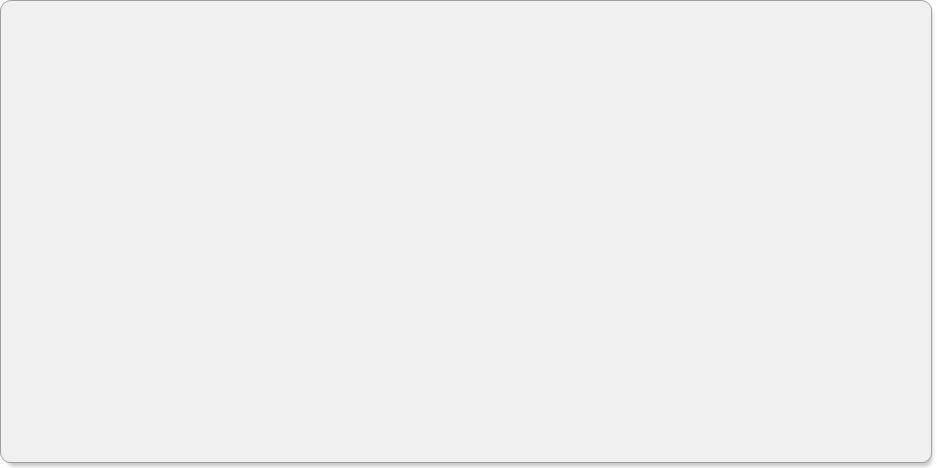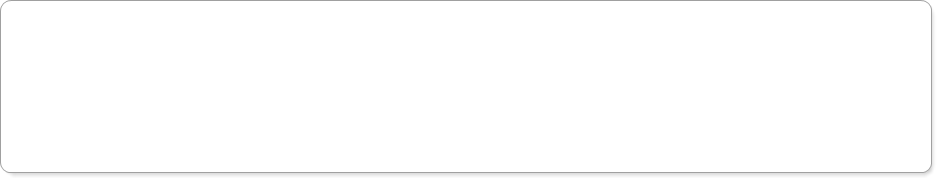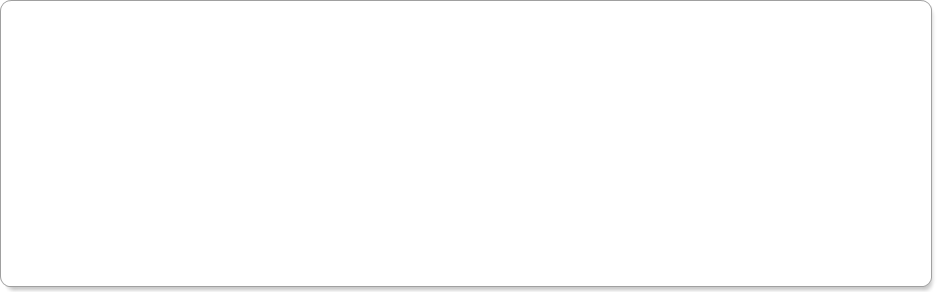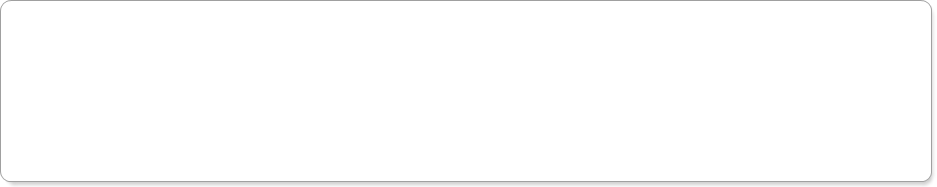Question 2:  For Question 1, suppose the object is located at 200 pixels from the top of the screen.  How far is the object from the top edge of the camera view, in units of feet?  Round your answer to three places after the decimal, and enter only a number, not units.
Question 3:  Suppose I have found the homogeneous transformation matrix from the base-frame (frame 0) to the camera frame.  How can I find the position of an object in the base-frame from the object position in the camera frame?
Question 4:  Suppose you have a camera mounted on a helicopter pointing straight down looking at a helipad.  The coordinate system on the helipad and the coordinate system on the camera is as shown.  What is the rotation matrix from the base-frame to the camera frame?
Question 5:  Suppose we've measured the distances between the base-frame and the camera frame as shown here.  What is the displacement vector from the base-frame to the camera frame?
Question 6:  For Questions 4 and 5, now suppose I know that the pixel-to-meter conversion is 640 pixels per 10 meters. I have found the location of a person as 100 pixels in the Xc direction and 300 pixels in the Yc direction.  Where is the person located in the Xc direction (that is, in the X direction in the CAMERA frame), in units of meters?  Round to three decimal places.
Question 7:  For Question 6, where is the person located in the Yc direction (that is, in the Y direction in the CAMERA frame), in units of meters?  Round to three decimal places.
Question 8:  For Questions 4-7, where is the person located in the X0 direction (that is, in the X direction in the BASE FRAME), in units of meters?  Round to three decimal places.
Question 9:  For Questions 4-7, where is the person located in the Y0 direction (that is, in the Y direction in the BASE FRAME), in units of meters?  Round to three decimal places.
Question 10: Suppose the base-frame and the camera frame are positioned as shown here.  Which displacement vector is correct?
Questions 11-15:  Suppose that you have a camera inclined relative to the field of view in the same way as illustrated in the video for today, but at a different angle of inclination.  Suppose you have collected the data shown here.  Fill in the centimeter locations (positions in  world coordinates) that correspond to the given X, Y pixel locations.  Round to 3 places after the decimal.
Question 1: Suppose you have a camera with a resolution of 1280x720, pointed at a wall.  You walk over to the wall and measure the area of the wall that can be seen by the camera as 6 feet high and 10 2/3 feet wide.  Then, you place an object on the wall and use background subtraction to find the location in camera coordinates.  When the object is located at 915 pixels in the X (width) direction, how far (in feet) is the object from the left edge of the camera view?  Round your answer to 3 places after the decimal, and enter only a number in the box; don't include units.
Add the homogeneous transformation matrix to the object position in the camera frame
Subtract the homogeneous transformation matrix from the object position in the camera frame
Multiply the homogeneous transformation matrix by the object position in the camera frame
Divide the homogeneous transformation matrix from the object position in the camera frame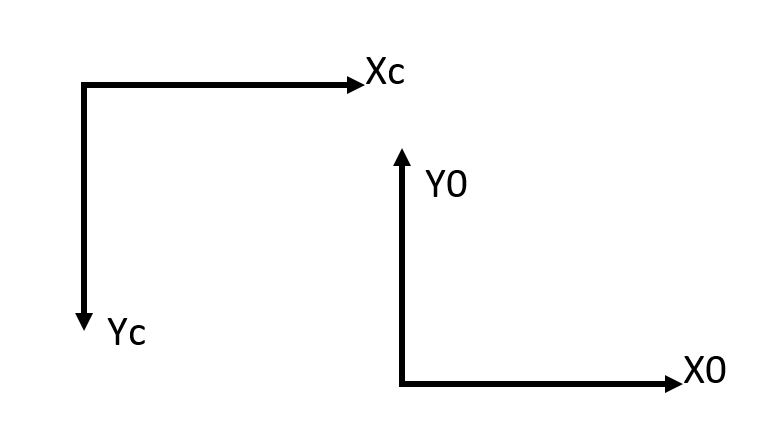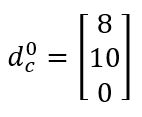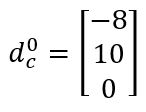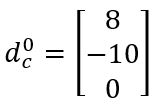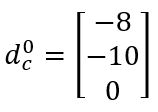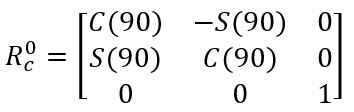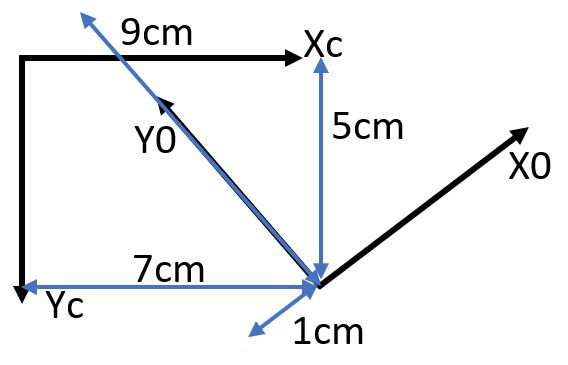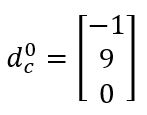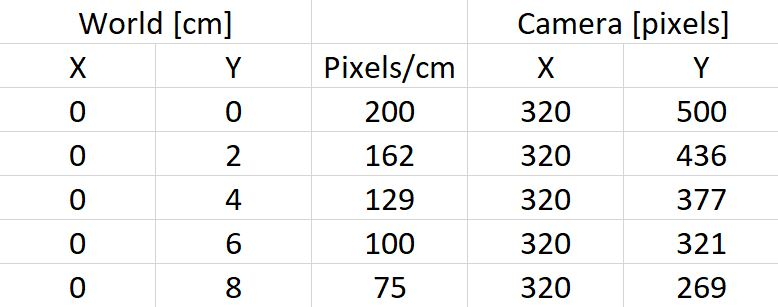Questions 11 and 12:
X = 150 pixels, Y = 100 pixels
X Position [cm]:
Y Position [cm]:
X Position [cm]:
Y Position [cm]:
Questions 13-14:
X = 400 pixels, Y = 200 pixels
Question 15: At which pixel value is the world coordinate Y = 10cm? Round to a whole number.